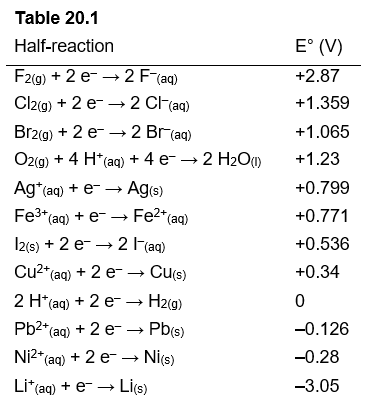# Problem: Using Table 20.1, which substance can oxidize I- (aq) to I2 (s)?A) Br2 (l)B) Cu2+ (aq)C) Ag (s)D) Br- (aq)E) Ni2+ (aq)

###### FREE Expert Solution

We’re being asked to determine the substance that can oxidize I-(aq) to I2(s)

Recall the mnemonics LEO GER.

Loss Electrons → Oxidation → Reducing Agent
Gain Electrons → Reduction → Oxidizing Agent

This means the element that gains electrons/reduced is the oxidizing agent while the element that loses electrons/oxidized is the reducing agent.

The ease to remove an electron from an element is also related to cell potential.###### Problem DetailsUsing Table 20.1, which substance can oxidize I- (aq) to I2 (s)?

A) Br2 (l)

B) Cu2+ (aq)

C) Ag (s)

D) Br- (aq)

E) Ni2+ (aq)